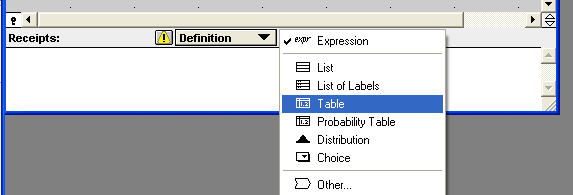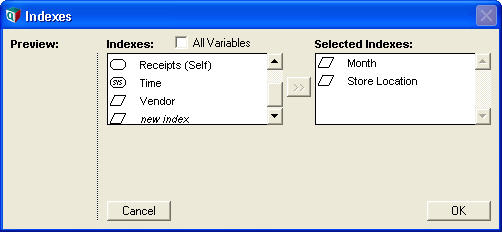# Table

## Table(I, J, K,...)(expr, expr2, expr3,...)

The table function defines an Edit Table -- a user-modifiable array of numbers, text, or expressions. The number of dimensions is specified by the number of indexes listed.

## Expression Syntax

The table function (along with the other table-like functions DetermTable, ProbTable, IntraTable) has an unusual syntax in that the function name is followed consecutively by parenthesized parameter lists.

The first parameter list enumerates the table indexes for the edit table. The keyword Self may appear as one of the indexes, with the self-index values being listed in the IndexVals attribute.

The second parameter list contains the cell contents. Each item is separated by a comma. The number of expressions in the second parameter list should be equal to the product of the number of elements in each index listed. The cells are defined in a manner such that the index listed first vary the slowest, while the index listed last varies fastest. Thus, in:

Table(I, J)(1, 2, 3, 4)

where

Index I := [1, 2]
Index J := ['a', 'b']

The elements would appear in the order:

[I = 1, J = 'a'], [I = 1, J =' b'], [I = 2, J = 'a'], [I = 2, J = 'b'].

If you were to reverse the order of the indexes in the first parameter list to:

Table(J, I)(1, 2, 3, 4)

Then the elements would appear in the order:

[I = 1, J = 'a'], [I = 2, J = 'a'], [I = 1, J = 'b'], [I = 2, J = 'b'].

When listing cell expressions, every expression must be a well-formed expression, so that the full table definition can be parsed.

## Creating a Table

To define a variable as an edit table (for details, see To make an edit table), select Table from the definition-type pulldown in the Attribute panel or Object window:Then, from the Index Chooser dialog, select the table indexes by moving the indexes to the right box:An edit table displays as a rectangular grid of cells, in which you can enter values into each cell.

## Table Splicing

One of the most powerful features in Analytica is Table Splicing, which ensures consistency of edit tables as their table indexes are changed. The Table Splicing feature is applied to definitions in which Table appears at the top level. If Table is used within a definition, the splicing feature is not applied. See Table Splicing for more information.

## Using Edit Tables

See Tutorial: Arrays in the Analytica Tutorial.

## Evaluation

When a table is evaluated, the expressions in all cells are evaluated, and the results are condensed back into a single array.

When a cell evaluation introduces an dimension not already present as a table index, the result will have that new dimension. Thus, the dimensionality of the result is the union of the table indexes and any dimensions introduced by cell evaluates.

When a cell evaluates to an array having one of the table dimensions as an array dimension, the value in the coordinate corresponding to that cell will have the value obtained by slicing the cell's result by the cell's coordinates.

A Table cannot contain expressions that refer to values in different cells of the same table. This would create a circular reference, which is disallowed. Since intra-table (or within-table) dependencies is very bad practice, leads to difficult to understand and maintain models, and to much potential for errors. However, if you need intra-cell dependencies, these are possible by using the IntraTable function, which is essentially the same as Table with this one difference that intra-cell dependencies are allowed (as long as the intra-cell dependencies don't contain a cycle between the cells). See IntraTable for more information.

The cells of an edit table can be OLE-linked from an external application such as an Excel spreadsheet. To establish the link, copy a block of cells matching the size of the table grid, then in Analytica select EditPaste Special... and select Paste Link. The data becomes linked to the original, such that when the data in the external application changes, these changes are instantaneously reflected in the edit table.

When the row (or column) index is defined as a list of numbers or list of labels, you can easily add, insert or delete rows (or columns), or change the value or label of index elements, directly from the edit table.

For example, to add a row, click on the last row-header cell and press the down arrow. To insert a row, click on the row header at the position for the new row and select Add Rows... from the Edit menu or right-mouse context menu.

## Browse Mode Editing

The cells of an edit table can be altered by model end-users from browse mode when the edit table has an associated input node. To set this up to allow editing, select the node with the table definition while in edit mode and select Object menuMake Input Node from the menu.

An index of an edit table can be edited from browse mode only when the index itself has an input node and is defined as a list of numbers or a list of labels.

## Default Values

The default cell value for an edit table is taken from the TableCellDefault attribute of the variable defined as a table. If that isn't specified, the value of the Sys_TableCellDefault system variable is used. The Analytica default for this system variable is 0, so that newly created cells are initialized with the number 0. The TableCellDefault value can be the identifier of another variable, which itself is defined as a Table (with a subset of the indexes). By doing so, the defaults table can provide different default values for each column of a table.

### Blank Cell Defaults

When elements are inserted into indexes that are used as table indexes, new values usually need to be specified for any newly inserted rows. Forgetting to specify some of these value can be an easy source of errors. To avoid these errors, you can configure your model (or an individual table) to use blank cells as the default. When evaluated, a table with blank cells will issue a warning. This can help to find inputs that would have otherwise been forgotten.

To configure for blank-cell defaults, enter the typescript window (press F12) and type:

Sys_TableCellDefault :